# Stein manifold

(diff) ← Older revision | Latest revision (diff) | Newer revision → (diff)

holomorphically-complete manifold

A paracompact complex analytic manifoldwith the following properties:

1) for any compact setthe set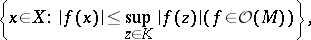where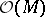is the algebra of holomorphic functions on, is compact (holomorphic convexity);

2) for any two different points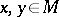there is a function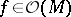such that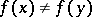(holomorphic separability);

3) in a neighbourhood of any point there is a holomorphic chart whose coordinate functions belong to.

The requirement of holomorphic convexity can be replaced by the following one: For any sequence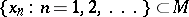without limit points there is a functionsuch that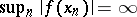.

The class of Stein manifolds was introduced by K. Stein  as a natural generalization of the notion of a domain of holomorphy in. Any closed analytic submanifold inis a Stein manifold; conversely, any-dimensional Stein manifold has a proper holomorphic imbedding in(cf. Proper morphism). Any non-compact Riemann surface is a Stein manifold. The direct generalization of a Stein manifold is a Stein space.